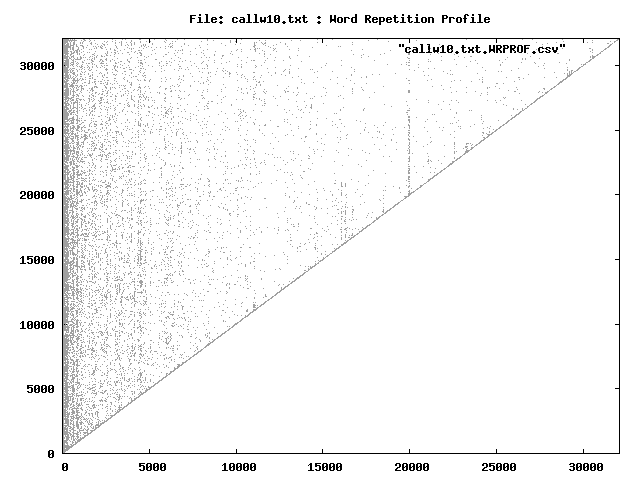Statistics on the text file callw10.txt, using the command:zwc -s -d -l -o outfiles -f callw10.txt The total word count is 32083 The unique words number 4783 The ratio unique/total is 14.9082 % The highest ranking 64 words make up 50.145 % of the total, OR 1.338 % of the unique words make up 50.145 % of the total volume The number of words used only once 2535 The ratio (words used only once)/total is 7.9014 % The average of the ratios between frequencies is 1.0018